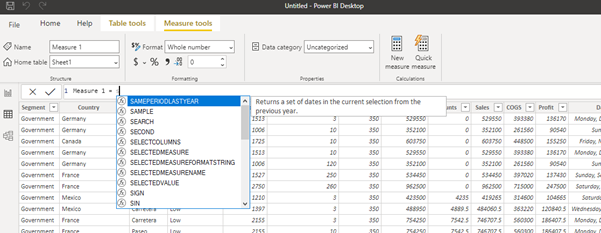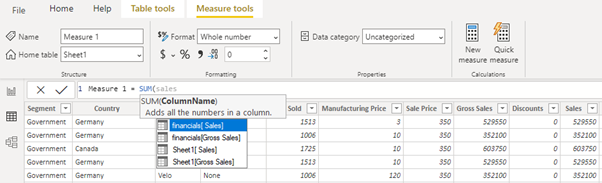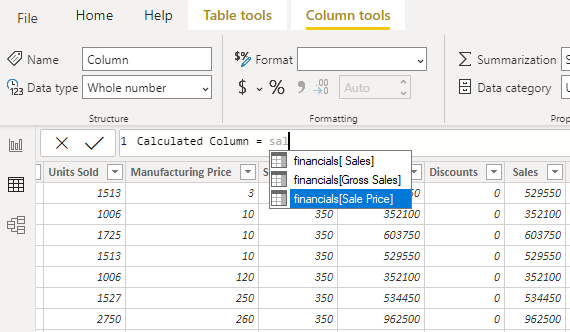# Measures vs Calculated Columns

There is a common mistake which a Power BI novice can perform while writing Dax measures which is related to row context and filter context. Measures are usually used for aggregation and calculated columns are used for row-wise calculations. So, if we are writing a Dax measure but haven’t made use any of aggregate function like sum or avg while writing the measure and are constantly stuck at a point where we don’t have options to select for column names then there is only one thing to remember, which is, when writing a measure we need to use the aggregate functions(Sum, Avg, Min, Max) for making our Dax measure usable and successful otherwise we can’t build a measure if we are not using these functions because measures work on a filter context. But in case of calculated columns we are not restricted or bounded to make use of aggregate functions because they work on row context basis evaluation so they provide column names without making use of these functions.